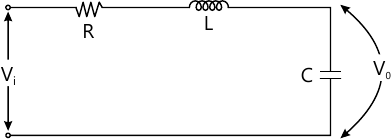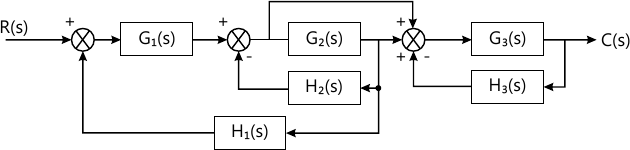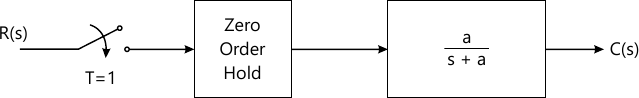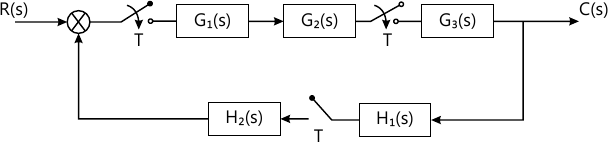Total marks: --
Total time: --
INSTRUCTIONS
(1) Assume appropriate data and state your reasons
(2) Marks are given to the right of every question
(3) Draw neat diagrams wherever necessary

Solve any one question from Q1 and Q2
1 (a) Consider the R-L-C network shown in Fig. 1:
i) Obtain transfer function if Vi and Vo are input and output voltage respectively.
ii) Find the location of poles in terms of R, L and C.
iii) If R=1 MΩ, C=1 μF, L=1 mH. Is the location of poles of transfer function given in (i) are real? If yes, find the location.6 M
1 (b) If $$G(s)= \dfrac {K}{s(s+64)}$$ with H(s)=1, determine value of K so that damping factor is 0.5. For this value of 'K' determine:
i) Rise time, and
ii) Settling time.
Assume unit step input.
6 M

2 (a) Find $$\dfrac {C(s)}{R(s)}$$ for the system shown in Fig. 2 using Block diagram rules.6 M
2 (b) The open loop transfer function of unity feedback system is:
$$G(s) = \dfrac {K}{s(\tau s+1)}, K, \tau > 0$$ with a given value of K, the peak overshoot was found to be 80%. Suppose peak overshoot is decreased to 20% by decreasing gain K. Find the new value of K (say K2) in terms of the old value.
6 M

Solve any one question from Q3 and Q4
3 (a) Comment on stability of a system using Routh's criteria, if characteristics equation is D(s)=s4+5s3+s2+10+1. How many poles lies in Right of s-plane?
4 M
3 (b) Construct Bode Plot and calculate GM, PM, Wgc and Wpc if $$G(s) = \dfrac {200(s+20)}{s(2s+1)(s+40)}$$ and H(s)=1.
8 M

4 (a) Open loop transfer function of unity feedback system is $$G(s) = \dfrac {K}{s(s+2)(s+10)}.$$ Sketch the complete root locus and comment on stability of system.
8 M
4 (b) For unity feedback system with $$G(s) = \dfrac {100}{s(s+5)}$$.
Determine:
i) Resonance peak
ii) Resonance frequency.
4 M

Solve any one question from Q5 and Q6
5 (a) Enlist any two advantages of state space approach over transfer function. Obtain a state space representation in controllable and observable canonical form for the system $$G(s) = \dfrac {s+3}{s^2 + 3s +2}$$
6 M
5 (b) Obtain the state space representation of system whose differential equation is: $\dfrac {d^2 y}{dt^3}+ 2 \dfrac {d^2 y}{dt^2}+ 3 \dfrac {dy}{dt}+ 6y = \dfrac {d^2u}{dt^2} - \dfrac {du}{dt}+ 2u.$ Also find controllability and observability of the system. Assume zero initial conditions.
7 M

6 (a) Obtain state transition matrix if: $i) \ \dfrac {dx}{dt} = \begin{bmatrix} 0 &1 \\-1 &0 \end{bmatrix}x \\ ii) \ \dfrac {dx}{dt} = \begin{bmatrix} 0 &1 \\0 &0 \end{bmatrix} x$ using Laplace transformation.
6 M
6 (b) Write a short note on 'state transition matrix and its properties'.
4 M

Solve any one question from Q7 and Q8
7 (a) Advantage of digital control system over analog control systems.
4 M
7 (b) Application of PLC (Programmable Logic Controller) in Elevator/List.
4 M
7 (c) PID controllers and its operational characteristics.
5 M

8 (a) Obtain pulse transfer function of the system shown in Fig. 3 with a=1.6 M
8 (b) Obtain pulse transfer function of system shown in Fig. 47 M

More question papers from Control Systems